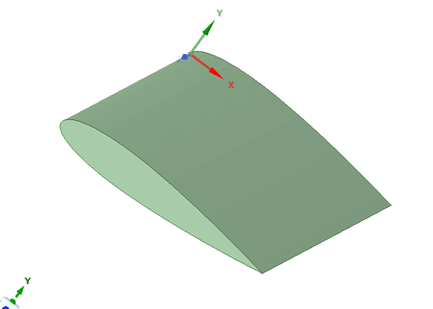# 如何在 DesignSpark Mechanical 中使用方程工具来绘制草图？

DesignSpark Mechanical V6.0

• 参数包括一个名称，并用方括号 [L] 括起来

• 函数包括一个函数名称，后跟包含参数值的括号  例如：Sine([t]-[r])

• 下表列出了常见的预定义内在函数

Abs

Acos

Asin

Atan

Cos

Sin

Sqrt

Tan

Exp

Log

Log10

Pow

x ([t])
y 5*([a])*((0.2969*Sqrt([t]))-(0.1260*[t])-(0.3516*Pow(([t]),2.0))+(0.2843*Pow(([t]),3.0))-(0.1036*Pow(([t]),4.0)))
z 0
Interval(t) Start:0, End:1, Scale:50
a 0.15

x ([t])
y -5*([a])*((0.2969*Sqrt([t]))-(0.1260*[t])-(0.3516*Pow(([t]),2.0))+(0.2843*Pow(([t]),3.0))-(0.1036*Pow(([t]),4.0)))
z 0
Interval(t) Start:0, End:1, Scale:50
a 0.15RS DesignSpark is the go-to platform for students, makers, hobbyists and professional design engineers, providing design resources such as the award winning DesignSpark PCB and DesignSpark Mechanical CAD software. Join the community today at https://www.rs-online.com/designspark/register